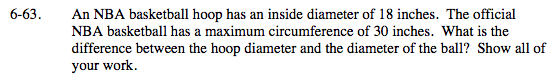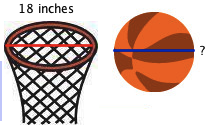### Home > MC2 > Chapter 6 > Lesson 6.1.6 > Problem6-63

6-63.Circumference = π · diameter
If an official NBA basketball has a circumference of 30 inches, what would its diameter be based on this formula?

$\text{Diameter } = \frac{30\text{ in}}{\pi} \approx 9.55 \text{ in}$

If 9.55 inches is the approximate diameter of a ball, what is the difference between the hoop and the ball diameter?